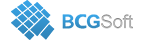BCGSuite for MFC
 virtual void CBCGPChartTrendFormula::GeneratePoints ( )
virtual

Builds an array of screen positions of non-discrete curve representing a formula on a diagram.

This method is called to generate screen positions of non-discrete curve representing a formula on a diagram.

If the Chart is "dirty" and the input series was specified, it recalculates coefficients for selected trend line type. Then it calculates a step by dividing the size of X axis in pixels by the range of X axis in logical units. This step is used to loop from minimum range up to maximum range and call OnCalculateYValue with the logical X value corresponding to each pixel on the axis.

Implements CBCGPChartBaseFormula.